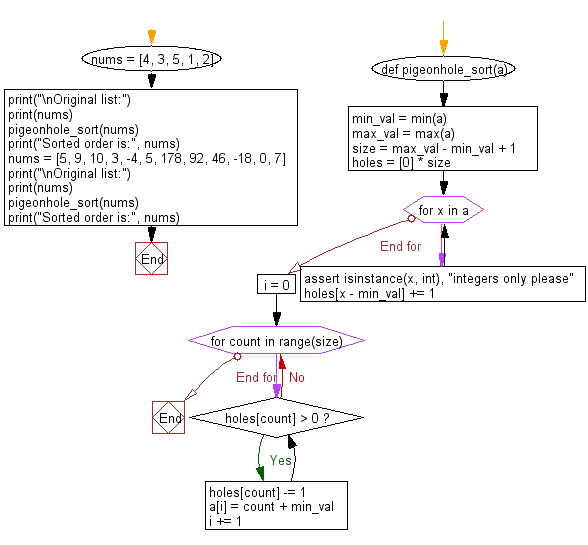﻿ Python: Sort unsorted numbers using Pigeonhole sorting - w3resource# Python: Sort unsorted numbers using Pigeonhole sorting

## Python Search and Sorting : Exercise-33 with Solution

Write a Python program to sort unsorted numbers using Pigeonhole sorting.

Pigeonhole sorting is a sorting algorithm that is suitable for sorting lists of elements where the number of elements (n) and the length of the range of possible key values (N) are approximately the same. It requires O(n + N) time. It is similar to counting sort, but differs in that it "moves items twice: once to the bucket array and again to the final destination [whereas] counting sort builds an auxiliary array then uses the array to compute each item's final destination and move the item there."
The pigeonhole algorithm works as follows:
1. Given an array of values to be sorted, set up an auxiliary array of initially empty "pigeonholes", one pigeonhole for each key in the range of the keys in the original array.
2. Going over the original array, put each value into the pigeonhole corresponding to its key, such that each pigeonhole eventually contains a list of all values with that key.
3. Iterate over the pigeonhole array in increasing order of keys, and for each pigeonhole, put its elements into the original array in increasing order.

Sample Solution:

Python Code:

``````#Ref. https://bit.ly/3olnZcd
def pigeonhole_sort(a):
# size of range of values in the list (ie, number of pigeonholes we need)
min_val = min(a)  # min() finds the minimum value
max_val = max(a)  # max() finds the maximum value
size = max_val - min_val + 1  # size is difference of max and min values plus one
# list of pigeonholes of size equal to the variable size
holes =  * size
# Populate the pigeonholes.
for x in a:
assert isinstance(x, int), "integers only please"
holes[x - min_val] += 1
# Putting the elements back into the array in an order.
i = 0
for count in range(size):
while holes[count] > 0:
holes[count] -= 1
a[i] = count + min_val
i += 1

nums = [4, 3, 5, 1, 2]
print("\nOriginal list:")
print(nums)
pigeonhole_sort(nums)
print("Sorted order is:", nums)
nums = [5, 9, 10, 3, -4, 5, 178, 92, 46, -18, 0, 7]
print("\nOriginal list:")
print(nums)
pigeonhole_sort(nums)
print("Sorted order is:", nums)
```
```

Sample Output:

```Original list:
[4, 3, 5, 1, 2]
Sorted order is: [1, 2, 3, 4, 5]

Original list:
[5, 9, 10, 3, -4, 5, 178, 92, 46, -18, 0, 7]
Sorted order is: [-18, -4, 0, 3, 5, 5, 7, 9, 10, 46, 92, 178]
```

Flowchart:Python Code Editor:

What is the difficulty level of this exercise?

Test your Programming skills with w3resource's quiz.

﻿

## Python: Tips of the Day

Use Reversed() In for Loops:

```>>> tasks = ['laundry', 'picking up kids', 'gardening', 'cooking']